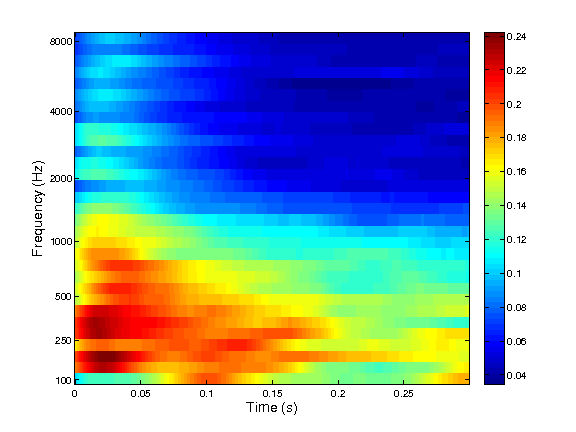THE AUDITORY MODELING TOOLBOX

Applies to version: 0.9.2

Go to function

BREEBAART2001PREPROC - Auditory model from Breebaart et. al. 2001

Usage

[outsig, fc] = breebaart2001preproc(insig,fs);
[outsig, fc] = breebaart2001preproc(insig,fs,...);

Input parameters

 insig input acoustic signal. fs sampling rate. tau characteristic delay in seconds (positive: left is leading) ild characteristic ILD in dB (positive: left is louder)

Description

breebaart2001preproc(insig,fs,tau,ild) computes the EI-cell representation of the signal insig sampled with a frequency of fs Hz as described in Breebaart (2001). The parameters tau and ild define the sensitivity of the EI-cell.

The input must have dimensions time $$\times$$ times  signal no.

The output has dimensions time $$\times$$ times  signal no.

[outsig,fc]=breebaart2001preproc(...) additionally returns the center frequencies of the filter bank.

The Breebaart 2001 model consists of the following stages:

1. a gammatone filter bank with 1-erb spaced filters.
2. an envelope extraction stage done by half-wave rectification followed by low-pass filtering to 770 Hz.
4. an excitation-inhibition (EI) cell model.

Parameters for auditoryfilterbank, ihcenvelope, adaptloop and eicell can be passed at the end of the line of input arguments.

Examples

The following code sets up a simple test example

% Setup parameters
fs      = 44100;            % Sampling rate
T       = 0.3;              % Duration
Spl1    = 75;               % SPL of input signal 1
Spl2    = 75;               % SPL of input signal 2
rho     = 0;                % normalized correlation of signals
tau     = 0;
ild     = 0;

% Generate signals:
t  = [0:1/fs:T];
n1 = setdbspl(randn(length(t),1),Spl1);
n2 = setdbspl(randn(length(t),1),Spl2);
x1 = n1*sqrt((1+rho)/2) + n2*sqrt((1-rho)/2);
x2 = n1*sqrt((1+rho)/2) - n2*sqrt((1-rho)/2);

% Run the model and plot it
[ei_map, fc] = breebaart2001preproc([x1,x2], fs, tau, ild);
plotfilterbank(ei_map,1,fc,fs,'audtick','lin');